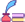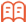##审稿期刊目次

## 期刊订阅

Open Access Article

Advances in International Applied Mathematics. 2022; 4: (1) ; 1-11 ; DOI: 10.12208/j.aam.20220001.

# Positive solutions to a class of singularelliptic systems一类奇异椭圆方程组的正解

*通讯作者： 申亚琳,单位：北京信息科技大学理学院 北京；

## Abstract

The goal of this paper is to study a class of elliptic systems with singular weight function. By using the fixed point index in a cone, we derive new results for the existence, nonexistence and multiplicity of positive radial solutions to this class of elliptic systems in different parameter intervals.

Key words： Singular Elliptic System;Positive Radial Solutions; Existence; Multiplicity; Nonexistence; Fixed point index; Cone

## 参考文献 References

 Joseph DD, Lundgren TS. Quasilinear dirichlet problems driven by positive sources[J]. Archive for Rational Mechanics & Analysis, 1973, 49: 241-269.

 Gelfand IM. Some problems in the theory of quasilinear equations[J]. Uspekhi Matematicheskikh Nauk, 1959, 14: 87-158.

 Coleman S, Glazer V, Martin A. Action minima among solutions to a class of Euclidean scalar field equations[J]. Communications in Mathematical Physics, 1978, 58: 211-221.

 Strauss WA. Existence of solitary waves in higher dimensions[J]. Communications in Mathematical Physics, 1977, 55: 149-162.

 Lions LP. Minimization problems in L1(R3) [J]. Journal of Functional Analysis, 1981, 41: 236-275.

 Gidas B, Ni WM, Nirenberg L. Symmetry and related properties via the maximum principle[J]. Communication in Mathematical Physics, 1979, 68: 209-243.

 Rey O. The role of the Green’s function in a nonlinear elliptic equation involving critical sobolev exponent[J]. Journal of Function Analysis, 1990, 89: 1-52.

 Lions LP. On the existence of positive solutions of semilinear elliptic equations[J]. Siam Review, 1982, 24: 441-467.

 Chang KC. Methods in Nonlinear Analysis[M]. Springer, 2005.

 Zhang Yichen, Feng Meiqiang. A coupled p-Laplacian elliptic system: Existence, uniqueness and asymptotic behavior[J]. Electronic Research Archive, 2020, 28: 1419-1438.

 Feng Meiqiang, Zhang Yichen. Positive solutions of singular multiparameter p-Laplacian elliptic systems[J]. Discrete & Continuous Dynamical Systems, 2022, 27: 1121-1147.

 Ma Ruyun. Existence of positive radial solutions for elliptic systems[J]. Journal of Mathematical Analysis and Applications, 1996, 201: 375-386.

 Hai D D.On a class of semilinear elliptic systems[J].Journal of Mathematical Analysis and Applications, 2003, 285: 477–486.

 Maya Chhetri a, Petr Girg bc.Existence of positive solutions for a class of superlinear semipositone systems[J].Journal of Mathematical Analysis and Applications, 2013, 408: 781-788.

 Cui Renhao, Li Ping, Shi Junping, Wang Yunwen. Existence,uniqueness and stability of positive solutions for a class of semilinear elliptic systems[J]. Topological Methods in Nonlinear Analysis, 2013, 42: 91-104.

 Peletier LA, Vandervorst RCAM. Existence and non-existence of positive solutions of non-linear elliptic systems and the biharmonic equation[J]. Differential and Integral Equations, 1992, 5: 747-767.

 Dalmasso Robert. Existence and uniqueness of positive solutions of semilinear elliptic systems[J]. Nonlinear Analysis, 2000, 39: 559-568.

 郭大钧. 非线性泛函分析[M]. 济南: 山东科学技术出版社, 1985.

 Dunninger DR,Wang Haiyan.Multiplicity of positive radial solutions for an elliptic system on an annulus[J].Nonlinear Analysis, 2000, 42: 803–811.